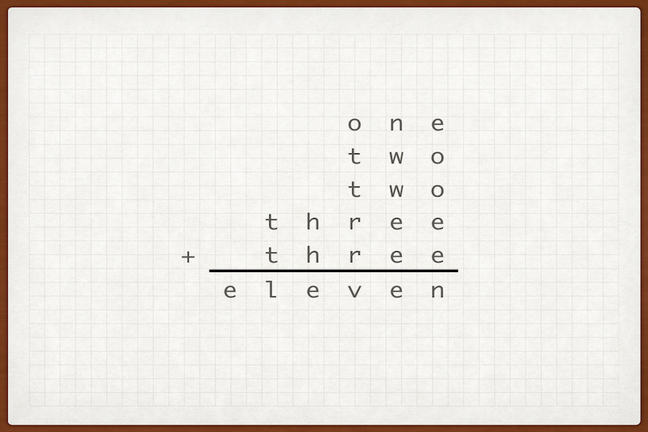## Want to keep learning?

This content is taken from the Davidson Institute of Science Education at the Weizmann Institute of Science's online course, Maths Puzzles: Cryptarithms, Symbologies and Secret Codes. Join the course to learn more.
1.16

## Davidson Institute of Science Education at the Weizmann Institute of ScienceDoubly true cryptarithm

# Different genres of cryptarithms

Up till now, we’ve taken a look at the basic cryptarithms. However, there is much more to cryptarithms than meets the eye! For instance, there exist different genres of cryptarithms.

# Double true

Double true cryptarithms are alphametics where the letters spell the words describing numbers, so that the actual wording is also true. In the example above, the cryptarithm is true, twice.

First, reading off the words, ‘one plus two plus two plus three plus three is indeed equal to eleven’.

Second, we can solve the cryptarithm, making the following substitutions:

E = 1, H = 4, L = 7, N = 9, O = 3, R = 6, T = 8, V = 2, W = 0

The cryptarithm is solved: 391 + 803 + 803 + 84611 + 84611 = 171219

Double true cryptarithms are solved the same way as regular alphametics.

# Roots, powers and division cryptarithms

Cryptarithms are not restricted to addition. We already saw some multiplication problems. Cryptarithms with other operations can also be fun. Here is a simple ‘power’ cryptarithm: $$(AA)^B = ABA$$ Let’s take a look at how to solve this one. First, we are looking for a 2-digit number (identical digits), which, when raised to a certain power, gives a 3-digit number. The smallest 2-digit number that is possible is 11. The power cannot be larger than 2 because then we get a 4-digit result. It cannot be smaller than 2 because then we get either 1 (when B=0) or a 2-digit number (AA when B=1). That’s all we need! The solution is $$11^2$$ where A=1 and B=2.

That was pretty straightforward, but if you get stuck, try looking for additional information. One thing we can do is to look at the units digit of the answer. In the case above, notice that when the number is raised to a certain power, the units digit in the result stays the same as the original units digit.

Square root cryptarithms can also be challenging. Remember that these can be reversed and turned into a multiplication problem. $$\sqrt{ABC}=BC$$ is the same as BCxBC=ABC

# Special words

Another fun genre is when the cryptarithms spell special words. Here is an example that Truman Collins has on his website:

SATURN + URANUS = PLANETS.

There are three possible solutions for this cryptarithm.

Truman has a lot of similar examples, and you can even generate your own ‘related words’ cryptarithms with his online cryptarithm generator. Other literary cryptarithms can be found on Mike Keith’s excellent website, but first I suggest you mark this step as complete, click ‘next’ and take this more advanced cryptarithm quiz.